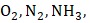# At 400 K, the root mean square (rms) speed of a gas(molecular weight = 40) is equal to the most probable speed of gasThe molecular weight of the gasis

## Question ID - 102463 :- At 400 K, the root mean square (rms) speed of a gas(molecular weight = 40) is equal to the most probable speed of gasThe molecular weight of the gasis

3537Next Question :
 The value of van der Waals constantfor the gasesandare 1.360, 1.390, 4.170, and, respectively. The gas which can most easily be liquefied is a)b)c)d)